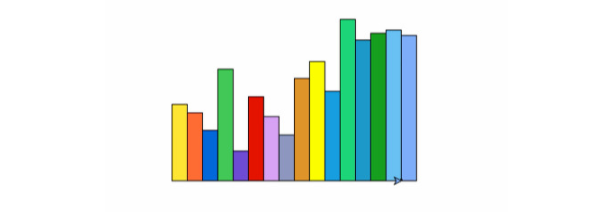### python制作简单动画_如何使用python制作简单的动画？

Python真的是无穷的好用，涉及内容非常广泛，比如接下来给大家介绍的制作动画，就可以利用python实现，相信小伙伴们听到这些内容肯定是非常感兴趣的，至于怎么去实现？大家想不想了解呢？下面小编就给大家整理出来了，一起来看吧~

import random as r

t.goto(x, y)        #画笔移到特定位置

t.fillcolor(c)       #设置填充颜色

t.begin_fill()

for i in range(2):  #画长方形

t.fd(20)

t.lt(90)

t.fd(h)

t.lt(90)

t.end_fill()for i in range(16):  #设置16中随机颜色

c.append((r.random(),r.random(),r.random()))

t.speed(11)         #绘图的速度设置为11

x = -180

t.tracer(False)     #关闭轨迹快速绘图

for i in range(16):

h = r.randint(20,220)

rect(h, c[i], x, 0)

x = x   20        #下一个长方形x坐标值x = -180

t.tracer(False)

for i in range(16):

h = r.randint(20,220)

rect(h, c[i], x, 0)

x = x   20

t.tracer(True)   #打开轨迹让绘制速度变慢

t.circle(1)         #画一个半径为1像素的圆，为了停留一段时间

t.tracer(False)

t.clear()

t.circle(1)

t.tracer(False)

t.clear()